## Summary

For me, one of the most interresting things to do with shaders is procedural images. To get started with that, we’re going to create a simple Checkerboard pattern.

This tutorial will build on the simple shader with only properties, but as always, you can also use the technique to generate colors in more complex shaders.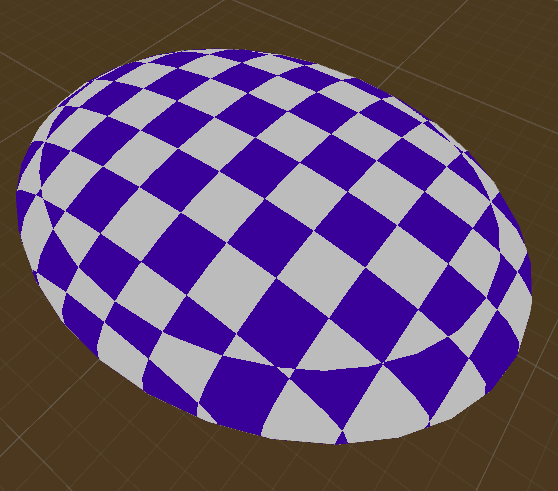## Stripes

I will take the world position of the surface to generate the chessboard texture, that way we can later move and rotate the model around and the generated patterns will fit together. If you want to pattern to move and rotate with the model, you can also use the object space coordinates (the ones from appdata, not multiplied with anything).

To use the worldposition in the fragment shader, we add the world position to the vertex to fragment struct and then generate the world position in the vertex shader and write it into the struct.

``````struct v2f{
float4 position : SV_POSITION;
float3 worldPos : TEXCOORD0;
}

v2f vert(appdata v){
v2f o;
//calculate the position in clip space to render the object
o.position = UnityObjectToClipPos(v.vertex);
//calculate the position of the vertex in the world
o.worldPos = mul(unity_ObjectToWorld, v.vertex);
return o;
}
``````

Then in the fragment shader, we can start by first doing a 1D chess field, so just alternating black and white lines. To do that, we take one of the axis of the position and modify the value. We start by flooring it. That means it’ll be the next smaller whole number. We do that to make sure we only have one color per unit.

Then we find out wether our field is a even or a odd one. To do that, we divide the value by two and take the fractional part (the part of the number after the dot). so now the even numbers are all 0(because after a division by 2 even numbers are still whole numbers, so their fractional part is 0) and all of the odd fields result in 0.5(because after a division by 2 odd numbers end up fractional, 1 becomes 0.5, 3 becomes 1.5…). To make the odd numbers white instead of grey, we can then multiply our value by 2.

``````fixed4 frag(v2f i) : SV_TARGET{
float chessboard = floor(i.worldPos.x);
//divide it by 2 and get the fractional part, resulting in a value of 0 for even and 0.5 for odd numbers.
chessboard = frac(chessboard * 0.5);
//multiply it by 2 to make odd values white instead of grey
chessboard *= 2;
return chessboard;
}
``````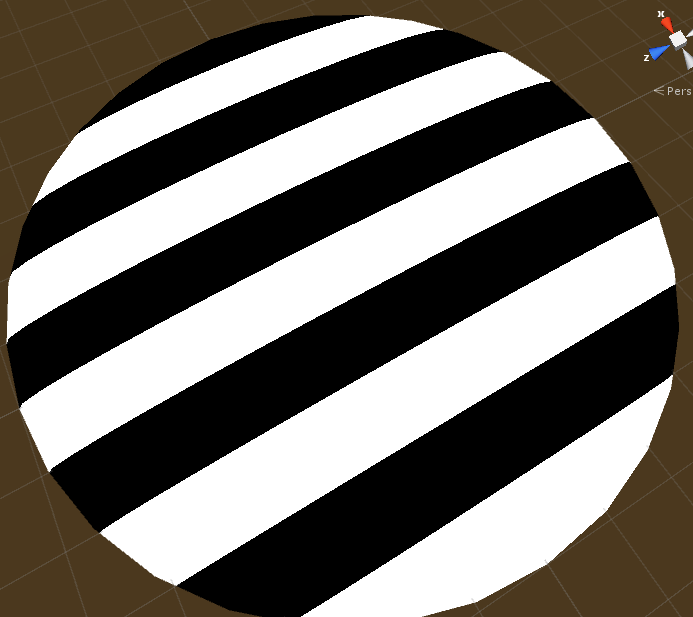## Checkerboard in 2d and 3d

Next, we make the pattern two dimensional. To do that we only have to add a additional axis to the value we’re evaluating. That’s because when we add one to our rows all of the even values become odd and the odd values become even. This is also the main reason why we floor our values. We easily could have made the pattern work in one dimension without flooring them, but this makes it easier to add more dimensions.

``````fixed4 frag(v2f i) : SV_TARGET{
float chessboard = floor(i.worldPos.x) + floor(i.worldPos.y);
//divide it by 2 and get the fractional part, resulting in a value of 0 for even and 0.5 for odd numbers.
chessboard = frac(chessboard * 0.5);
//multiply it by 2 to make odd values white instead of grey
chessboard *= 2;
return chessboard;
}
``````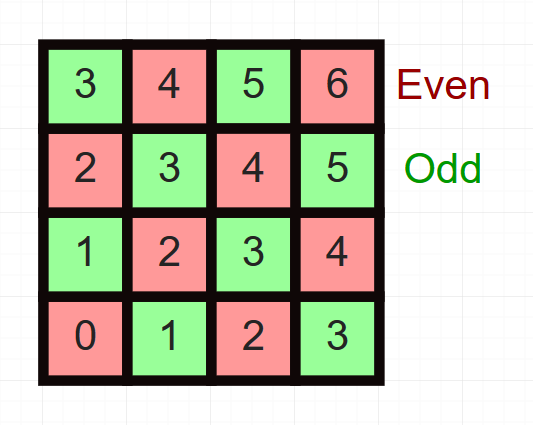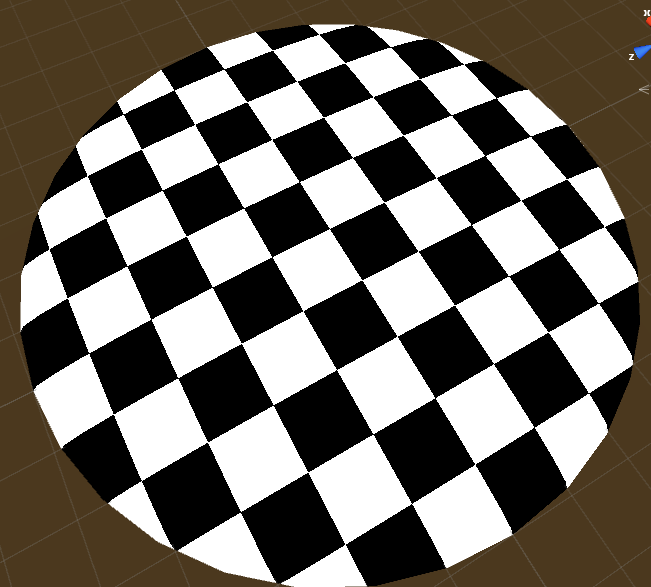After that we can go even further and add the third dimension in the same way as we added the second.

``````fixed4 frag(v2f i) : SV_TARGET{
float chessboard = floor(i.worldPos.x) + floor(i.worldPos.y) + floor(i.worldPos.z);
//divide it by 2 and get the fractional part, resulting in a value of 0 for even and 0.5 for odd numbers.
chessboard = frac(chessboard * 0.5);
//multiply it by 2 to make odd values white instead of grey
chessboard *= 2;
return chessboard;
}
``````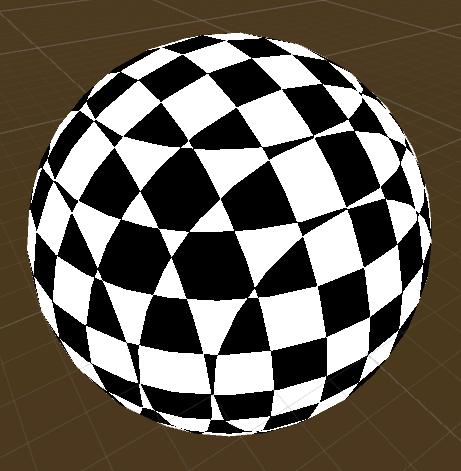## Scaling

Next I’d like to add the ability to make the pattern bigger or smaller. For that, we add a new property for the scale of the pattern. We divide the position by the scale before we do anything else with it, that way, if the scale is smaller than one, the pattern is generated as if the object is bigger than it is and as such it has more pattern density per surface area.

Another small change I made is that we now use floor on the whole vector instead of the components separately. That doesn’t change anything, I just think it’s nicer to read.

``````//...

//show values to edit in inspector
Properties{
_Scale ("Pattern Size", Range(0,10)) = 1
}

//...

float _Scale;

//...

fixed4 frag(v2f i) : SV_TARGET{
//scale the position to adjust for shader input and floor the values so we have whole numbers
float3 adjustedWorldPos = floor(i.worldPos / _Scale);
//divide it by 2 and get the fractional part, resulting in a value of 0 for even and 0.5 for off numbers.
chessboard = frac(chessboard * 0.5);
//multiply it by 2 to make odd values white instead of grey
chessboard *= 2;
return chessboard;
}

//...
``````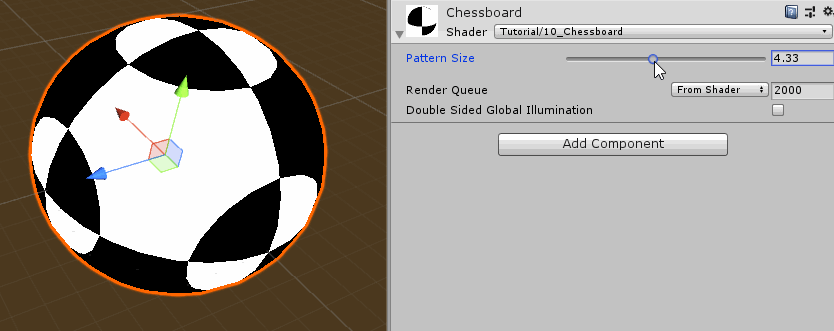## Customizable Colors

Finally I’d like to add the possibility to add Colors to the Pattern, One for the even areas, one for the odd. We add two new Properties and the matching values for those colors to the shader.

Then at the end of our fragment shader, we do a linear interpolation between the two colors. Since we only have two different values (zero and one), we can expect the interpolation to return either the color it interpolates from(for a input of 0) or the color it interpolates towards(for a input of 1). (If you’re confused by the interpolation, I explain it more thouroghly in another tutorial.

``````//...

//show values to edit in inspector
Properties{
_Scale ("Pattern Size", Range(0,10)) = 1
_EvenColor("Color 1", Color) = (0,0,0,1)
_OddColor("Color 2", Color) = (1,1,1,1)
}

//...

float4 _EvenColor;
float4 _OddColor;

//...

fixed4 frag(v2f i) : SV_TARGET{
//scale the position to adjust for shader input and floor the values so we have whole numbers
float3 adjustedWorldPos = floor(i.worldPos / _Scale);
//divide it by 2 and get the fractional part, resulting in a value of 0 for even and 0.5 for off numbers.
chessboard = frac(chessboard * 0.5);
//multiply it by 2 to make odd values white instead of grey
chessboard *= 2;

//interpolate between color for even fields (0) and color for odd fields (1)
float4 color = lerp(_EvenColor, _OddColor, chessboard);
return color;
}

//...
``````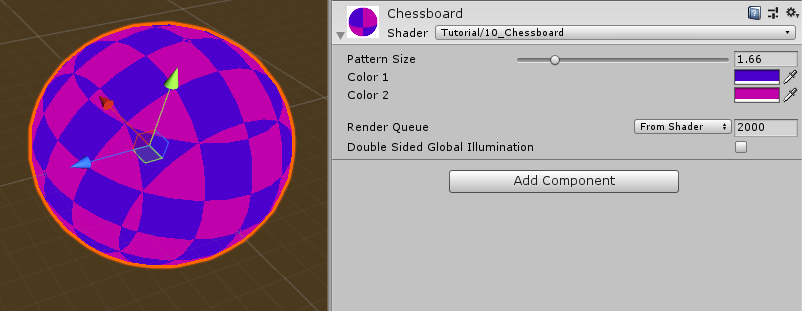The complete shader for interpolating generating a checkerboard pattern on a surface should now look like this:

``````Shader "Tutorial/011_Chessboard"
{
//show values to edit in inspector
Properties{
_Scale ("Pattern Size", Range(0,10)) = 1
_EvenColor("Color 1", Color) = (0,0,0,1)
_OddColor("Color 2", Color) = (1,1,1,1)
}

//the material is completely non-transparent and is rendered at the same time as the other opaque geometry
Tags{ "RenderType"="Opaque" "Queue"="Geometry"}

Pass{
CGPROGRAM
#include "UnityCG.cginc"

#pragma vertex vert
#pragma fragment frag

float _Scale;

float4 _EvenColor;
float4 _OddColor;

struct appdata{
float4 vertex : POSITION;
};

struct v2f{
float4 position : SV_POSITION;
float3 worldPos : TEXCOORD0;
};

v2f vert(appdata v){
v2f o;
//calculate the position in clip space to render the object
o.position = UnityObjectToClipPos(v.vertex);
//calculate the position of the vertex in the world
o.worldPos = mul(unity_ObjectToWorld, v.vertex);
return o;
}

fixed4 frag(v2f i) : SV_TARGET{
//scale the position to adjust for shader input and floor the values so we have whole numbers
float3 adjustedWorldPos = floor(i.worldPos / _Scale);
//divide it by 2 and get the fractional part, resulting in a value of 0 for even and 0.5 for off numbers.
chessboard = frac(chessboard * 0.5);
//multiply it by 2 to make odd values white instead of grey
chessboard *= 2;

//interpolate between color for even fields (0) and color for odd fields (1)
float4 color = lerp(_EvenColor, _OddColor, chessboard);
return color;
}

ENDCG
}
}